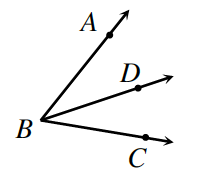Home > CCG > Chapter 6 > Lesson 6.2.4 > Problem6-85

6-85.In the diagram at right, $\overrightarrow{BD}$ bisects $∠ABC$. This means that $\overrightarrow{BD}$ divides the angle into two equal parts. If $m∠ABD=3x+24º$ and if $m∠CBD = 5x + 2º$ solve for $x$. Then find $m∠ABC$.

If $\overline{BD}$ divides $\angle ABC$ into two equal parts, what can you do with the equations for their measures?

$3x+24º=5x+2º$

Once you have solved for $x$, substitute it back into the expressions of each angle.
How can you use those measures to find the measure of $∠ABC$?